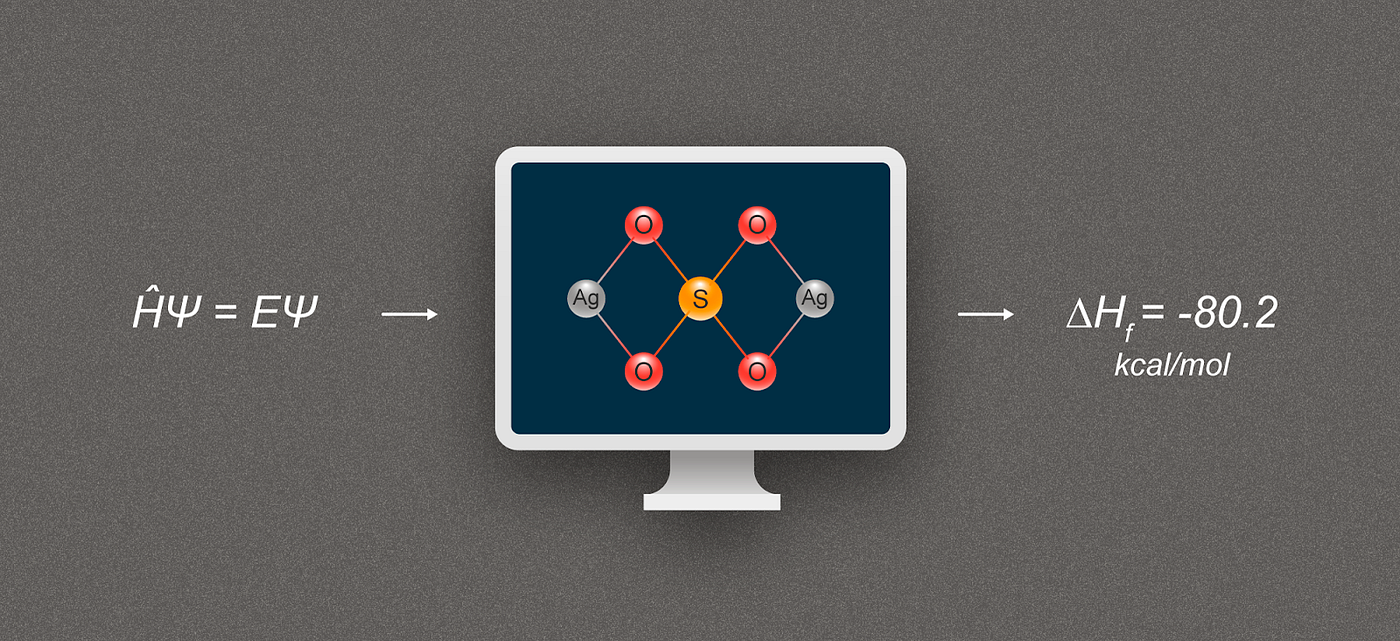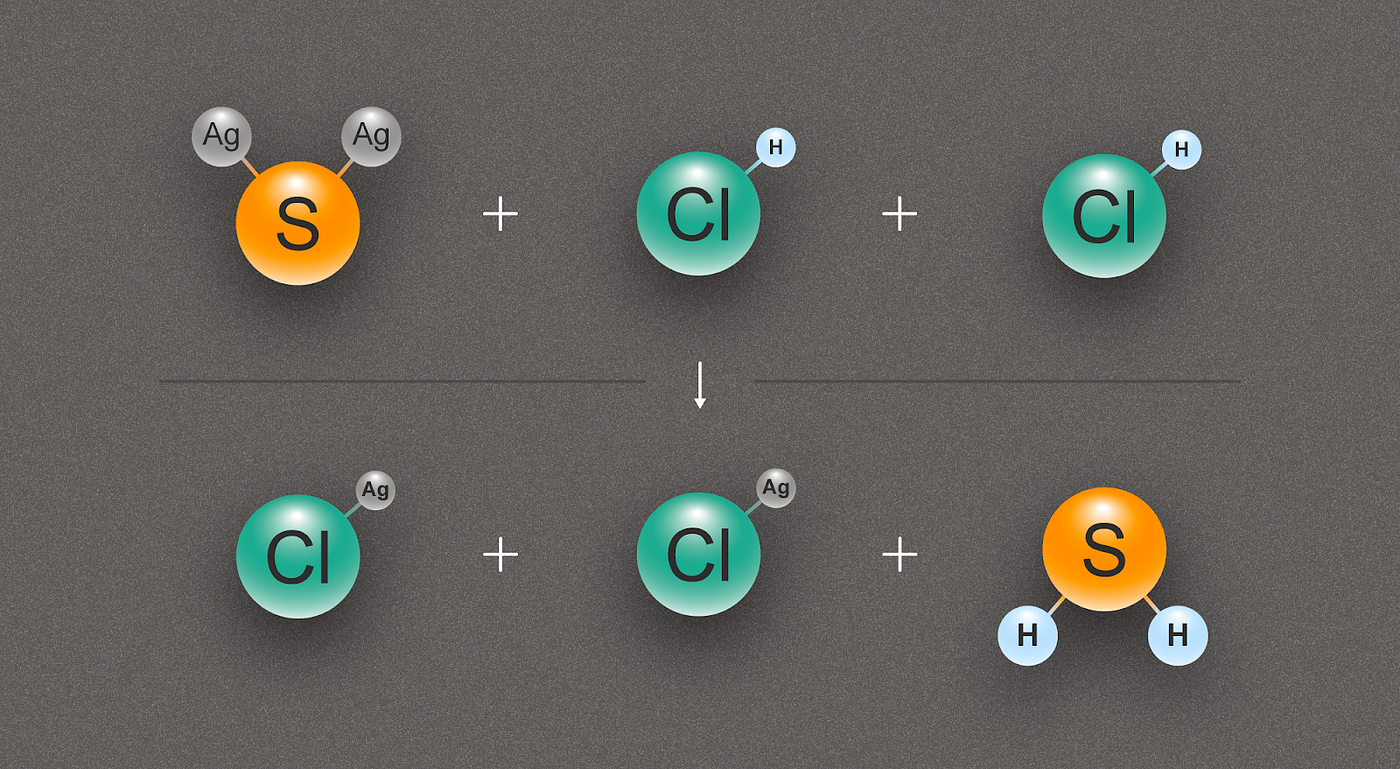# Researchers enrich silver chemistry

Researchers from the Moscow Institute of Physics and Technology have teamed up with colleagues in Russia and Saudi Arabia and proposed an efficient method for obtaining fundamental data necessary for understanding chemical and physical processes involving substances in the gaseous state. The proposed numerical protocol predicts the thermal effect of gas-phase formation of silver compounds and their absolute entropy. This includes first-ever such data for over 90 compounds. in the journal Inorganic Chemistry, the findings are important for the practical applications of substances containing silver: in water and wound disinfection, photography, rainmaking via cloud seeding, etc.

The team derived the precise values of two key characteristics — the enthalpy of formation and the entropy — of numerous silver compounds. The (from Greek “thalpein,” meaning “to heat”) of a system describes its state in terms of the energy of the constituent particles, pressure, and volume. According to , by multiplying stoichiometric coefficients and the difference between the formation enthalpies of the reactants and those of the products, one gets the amount of heat generated or consumed in a chemical reaction. Entropy is a measure of how disordered a system is. The second law of thermodynamics states that a system can spontaneously adopt a less organized state, so entropy grows with time.

Knowing the values of enthalpy and entropy is crucial for predicting whether a reaction will ever occur at given conditions. These characteristics also indicate how reaction yield and selectivity — the ratio between products — vary with temperature and pressure, allowing for optimization. The findings enable researchers to make predictions concerning chemical processes occurring in the gas phase. The data will also help manage the processes involved in thin film and pure sample deposition from the gas phase.

There are basically two ways for determining enthalpy and entropy changes: either through complex and costly experiments or by using the data from reference books and doing some arithmetic based on Hess’ law.

“The choice seems to be obvious, more so considering that you cannot experimentally measure the heat of some reactions,” said Yury Minenkov, senior researcher at the Laboratory of Supercomputing Methods in Condensed Matter Physics. “For example, incomplete graphite combustion always yields both carbon monoxide and carbon dioxide. So even by measuring the thermal effect of the reaction we could not determine carbon monoxide formation enthalpy.

“But the computational approach faces some problems,” Minenkov went on. “First, the enthalpies of formation and entropies are not known for every compound. Second, even if the data are available, no one can guarantee their accuracy. The values vary widely between reference books. At times, the measurement errors may be quite large.”

Luckily, quantum chemistry helps obtain the entropy and, to some extent, the enthalpy data. Each constituent molecule of a gaseous substance can be viewed as a system of positively charged nuclei and negatively charged electrons. Researchers can then apply electronic structure calculation methods to solve the molecular Schrödinger equation. This reveals the total electronic energy of the molecule, its wave function, and the spatial configuration of nuclei — that is, its 3D geometric structure. Physicists can then calculate the entropy and enthalpy of an ideal gas composed of such molecules. The entropy values obtained in this way are then introduced into reference books and used in thermodynamic calculations.

The problem with enthalpy is that, not being a fundamental value at this point, it significantly depends on the chosen method for Schrödinger equation calculation.Figure 1. Simplified diagram illustrating formation enthalpy calculation for silver compounds. The compound depicted is silver sulfate, Ag₂SO₄. Credit: @tsarcyanide/MIPT Press Office

Atomization reactions are usually employed to calculate the enthalpy of formation. In such reactions, the compound of interest breaks down into individual atoms. For example, silver sulfide — Ag₂S — yields one sulfur and two silver atoms. Since the enthalpies of formation of atomic substances are well-known and reported in reference books, it is possible to calculate the enthalpy of formation of the initial substance — in this case, silver sulfide — by finding the enthalpy change in the reaction via quantum chemistry methods.

However, when molecules composed of many atoms undergo atomization, this affects the electronic structure to such an extent that enthalpy, too, is significantly changed. The currently available methods of theoretical chemistry cannot account for these effects with enough accuracy.

The team of researchers from MIPT, the Frumkin Institute of Physical Chemistry and Electrochemistry of the Russian Academy of Sciences, Ivanovo State University of Chemistry and Technology, and Saudi Arabia’s King Abdullah University of Science and Technology has published a series of papers proposing a way to calculate the thermodynamic characteristics of and compounds with more accuracy.

In the case of silver sulfide, the researchers found its enthalpy of formation from the reaction with hydrochloric acid, which yields silver chloride and hydrogen sulfide (fig. 2). Since the number of bonds in the top row is the same as in the bottom row, the change in energy can be calculated with the least error.Figure 2. A diagram illustrating the chemical reaction between one silver sulfide (Ag₂S) and two hydrochloric acid (HCl) molecules, producing two molecules of silver chloride (AgCl) and one of hydrogen sulfide (H₂S). Credit: @tsarcyanide/MIPT Press Office

The heats of formation for silver chloride, hydrogen sulfide, and hydrochloric acid are known with a high accuracy, and computer modeling reveals the thermal effect of the reaction. From these data, one can derive the heat of formation of silver sulfide using Hess’ law.

“The classic coupled-cluster approach, known as CCSD(T), is the gold standard for solving the electronic Schrödinger equation in modern quantum chemistry,” Minenkov explained. “We replaced it with the local version called DLPNO-CCSD(T), which was developed not long ago at Max Planck Institute. This shrank the required computing power by an order of magnitude. Under the conventional CCSD(T), the computation time varies with the size N of the molecule as N⁷, so it is not an option for large molecules. The local version is much less time- and resource consuming.”

The team began by checking how well the results of their quantum chemical calculations agreed with the experimental thermodynamic and structural data. Reference books reported the values for 10 silver compounds, and they were a good match. Having thus ensured that their model is adequate, the researchers calculated the thermodynamic characteristics for 90 silver compounds missing in the books. The data are of use to both scientists working in the field of silver chemistry and for testing and calibrating new methods of electronic structure calculation.

Originally published at .

--

--